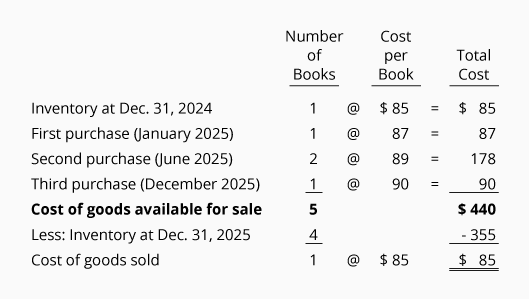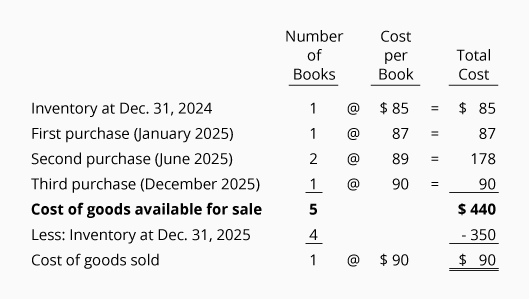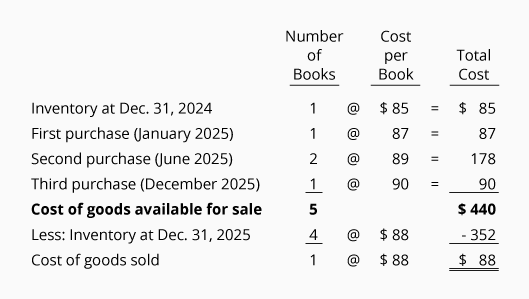# Periodic FIFO

Periodic means that the Inventory account is not routinely updated during the accounting period. Instead, the cost of merchandise purchased from suppliers is debited to the general ledger account Purchases. At the end of the accounting year the Inventory account is adjusted to equal the cost of the merchandise that has not been sold.

The cost of goods sold (which is reported on the income statement) is computed by taking the cost of the goods available for sale and subtracting the cost of the ending inventory.

FIFO is an acronym for first in, first out. Under the FIFO cost flow assumption, the first (oldest) costs are the first costs to leave inventory and be reported as the cost of goods sold on the income statement. The last (or recent) costs will remain in inventory and be reported as inventory on the balance sheet.

Remember that the costs can flow differently than the physical flow of the goods. For example, if the Corner Bookstore uses the FIFO cost flow assumption, the owner may sell any copy of the book but report the cost of goods at the first/oldest cost as shown in the exhibit that follows.

Let's demonstrate periodic FIFO with the following information from the Corner Bookstore:As before, we need to account for the cost of goods available for sale (5 books having a total cost of \$440). With FIFO we assign the first cost of \$85 to be the cost of goods sold. The remaining \$355 (\$440 - \$85) will be the cost of the ending inventory. The \$355 of inventory costs consists of \$87 + \$89 + \$89 + \$90. The \$85 cost that was assigned to the book sold is permanently gone from inventory.

If Corner Bookstore sells the textbook for \$110, its gross profit using periodic FIFO will be \$25 (\$110 - \$85). If the costs of textbooks continue to increase, FIFO will always result in more gross profit than other cost flows, because the first cost will always be lower.

## Periodic LIFO

Periodic means that the Inventory account is not updated during the accounting period. Instead, the cost of merchandise purchased from suppliers is debited to the general ledger account Purchases. At the end of the accounting year the Inventory account is adjusted to the cost of the merchandise that is unsold. The remainder of the cost of goods available is reported on the income statement as the cost of goods sold.

LIFO is an acronym for last in, first out. Under the LIFO cost flow assumption, the latest (or most recent) costs are the first ones to leave inventory and become the cost of goods sold on the income statement. The first/oldest costs will remain in inventory and will be reported as the cost of the ending inventory on the balance sheet.

Remember that the costs can flow differently than the goods. In other words, if Corner Bookstore uses periodic LIFO, the owner may sell the oldest (first) copy of the book to a customer, and report the cost of goods sold of \$90 (the cost of the most recently purchased book).

It's important to note that under periodic LIFO (not perpetual LIFO) you wait until the entire year is over before assigning the costs. Then you flow out of inventory the year's most recent costs first, even if those goods arrived after the last sale of the year. For example, assume the last sale of the year at the Corner Bookstore occurred on December 27. Also assume that the store's last purchase of the year arrived on December 31. Under periodic LIFO, the cost of the book purchased on December 31 is removed from inventory and sent to the cost of goods sold first, even though it was physically impossible for that book to be the one sold on December 27. (This reinforces our earlier statements that the flow of costs does not have to correspond with the physical flow of units.)

Let's illustrate periodic LIFO by using the data for the Corner Shelf Bookstore:As before we need to account for the cost of goods available for sale: 5 books having a total cost of \$440. Under periodic LIFO we assign the last cost of \$90 to the book that was sold. (If two books were sold, \$90 would be assigned to the first book and \$89 to the second book.) The remaining \$350 (\$440 - \$90) is reported as the cost of the ending inventory. The \$350 of inventory cost consists of \$85 + \$87 + \$89 + \$89. The \$90 assigned to the book that was sold is permanently gone from inventory.

If the bookstore sold the textbook for \$110, its gross profit using periodic LIFO will be \$20 (\$110 - \$90). If the costs of textbooks continue to increase, periodic LIFO will always result in the least amount of profit. The reason is that the last costs will always be higher than the first costs. Higher costs result in less profits and often lower income taxes.

## Periodic Average

When the periodic inventory system is used, the Inventory account is not updated and purchases of merchandise are recorded in the general ledger account Purchases.

With the average or weighted average cost flow assumption an average cost is calculated using the cost of goods available for sale (cost from the beginning inventory plus the costs of all the purchases made during the year). This means that the periodic average cost is calculated after the year is over—after all the purchases for the year have occurred. This average cost is then applied to the units sold during the year and to the units in inventory at the end of the year.

We will assume the same facts. There were 5 books available for sale for the year 2022 and the cost of the goods available was \$440. The weighted average cost of the books is \$88 (\$440 of cost of goods available ÷ 5 books). The average cost of \$88 is used to compute both the cost of goods sold and the cost of the ending inventory.Since the bookstore sold only one book, the cost of goods sold is \$88 (1 x \$88). The ending inventory of four unsold books is reported at the cost of \$352 (4 x \$88) . The total of the cost of goods sold plus the cost of the inventory should equal the cost of goods available (\$88 + \$352 = \$440).

If Corner Bookstore sells the textbook for \$110, its gross profit using the periodic average method will be \$22 (\$110 - \$88). This gross profit of \$22 lies between the \$25 computed using the periodic FIFO and the \$20 computed using the periodic LIFO.